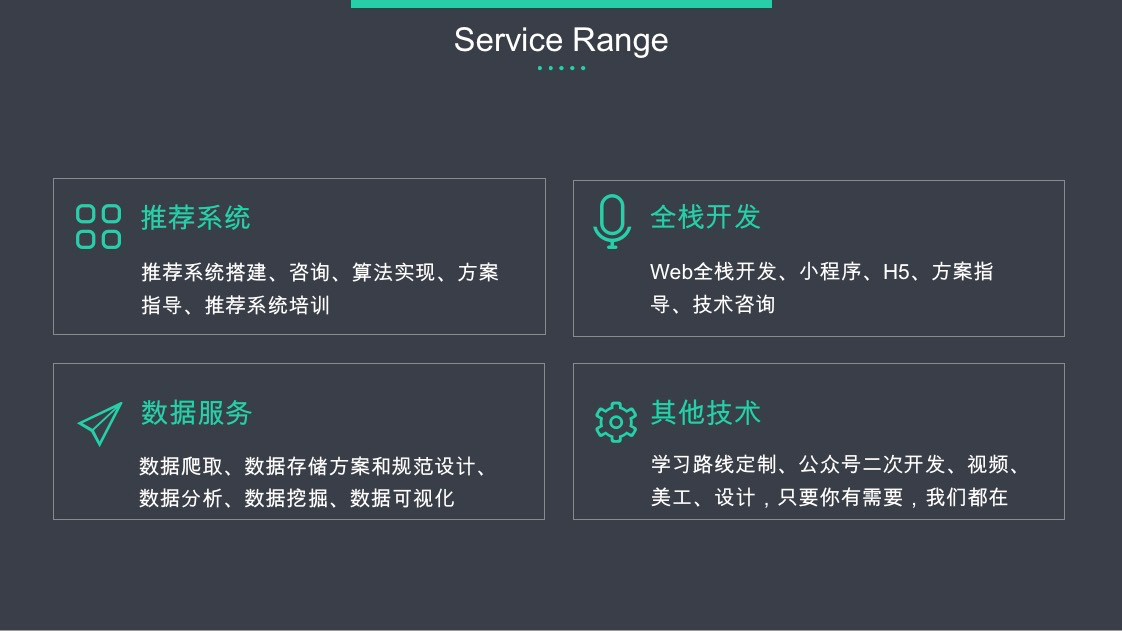# 简介

$\textstyle x$ 的维度为 \textstyle n+1，其中 \textstyle x_0 = 1 对应截距项 。） 由于 logistic 回归是针对二分类问题的，因此类标记 $y^{(i)} \in \{0,1\}$。假设函数(hypothesis function) 如下：

# 代价函数

$\textstyle 1\{ 值为真的表达式 \textstyle \}=1$

，$\textstyle 1\{ 值为假的表达式 \textstyle \}=0$。举例来说，表达式$\textstyle 1\{2+2=4\}$的值为1 ，$\textstyle 1\{1+1=5\}$的值为 0。我们的代价函数为：

# Softmax回归模型参数化的特点

Softmax 回归有一个不寻常的特点：它有一个“冗余”的参数集。为了便于阐述这一特点，假设我们从参数向量 $\textstyle \theta_j$中减去了向量 $\textstyle \psi$，这时，每一个$\textstyle \theta_j$都变成了 $\textstyle \theta_j - \psi(\textstyle j=1, \ldots, k)$。此时假设函数变成了以下的式子：

# 权重衰减

\begin{align}
h_\theta(x) &=
\frac{1}{ e^{\theta_1^Tx} + e^{ \theta_2^T x^{(i)} } }
\begin{bmatrix}
e^{ \theta_1^T x } \\
e^{ \theta_2^T x }
\end{bmatrix}
\end{align}

\begin{align}
h(x) &=
\frac{1}{ e^{\vec{0}^Tx} + e^{ (\theta_2-\theta_1)^T x^{(i)} } }
\begin{bmatrix}
e^{ \vec{0}^T x } \\
e^{ (\theta_2-\theta_1)^T x }
\end{bmatrix} \\
&=
\begin{bmatrix}
\frac{1}{ 1 + e^{ (\theta_2-\theta_1)^T x^{(i)} } } \\
\frac{e^{ (\theta_2-\theta_1)^T x }}{ 1 + e^{ (\theta_2-\theta_1)^T x^{(i)} } }
\end{bmatrix} \\
&=
\begin{bmatrix}
\frac{1}{ 1 + e^{ (\theta_2-\theta_1)^T x^{(i)} } } \\
1 - \frac{1}{ 1 + e^{ (\theta_2-\theta_1)^T x^{(i)} } } \\
\end{bmatrix}
\end{align}



# 中英文对照

• Softmax回归 Softmax Regression
• 有监督学习 supervised learning
• 无监督学习 unsupervised learning
• 深度学习 deep learning
• logistic回归 logistic regression
• 截距项 intercept term
• 二元分类 binary classification
• 类型标记 class labels
• 估值函数/估计值 hypothesis
• 代价函数 cost function
• 多元分类 multi-class classification
• 权重衰减 weight decay

【技术服务】，详情点击查看： https://mp.weixin.qq.com/s/PtX9ukKRBmazAWARprGIAg